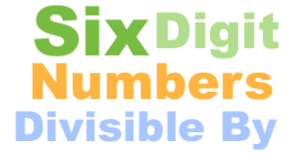Six digit numbers divisible by 12Six digit numbers (6-digit numbers) are numbers that have six digits in them. They range from 100000 to 999999. Therefore, there are a total of 900000 6-digit numbers.

If you divide a six digit number by 12 and you get a whole number with no remainder, then the 6-digit number is divisible by 12.

How many six digit numbers are divisible by 12?
Yes, we counted all the 6-digit numbers divisible by 12. There are 75000 six digit numbers divisible by 12. Out of the 75000 6-digit numbers divisible by 12, 75000 are even numbers and 0 are odd numbers.

What is the smallest six digit number divisible by 12?
The smallest 6-digit number divisible by 12 is 100008. This is sometimes also referred to as the first six digit number divisible by 12 or the lowest 6-digit number divisible by 12.

What is the largest six digit number divisible by 12?
The largest 6-digit number divisible by 12 is 999996. This is sometimes also referred to as the last six digit number divisible by 12 or the greatest 6-digit number divisible by 12.

List of all six digit numbers divisible by 12
The list of all 6-digit numbers divisible by 12 starts with 100008 and grows in intervals of 12 to 999996. Due to size constraint, we cannot list all 6-digit numbers divisible by 12. However, we have shortened the list to show the beginning and the end of the list so you can see the trend and fill in the blanks.

100008 100020 1100032 . . . 999996

That's it everyone. We hope you found our "Six digit numbers divisible by 12" information helpful.

Six Digit Numbers Divisible By Calculator
Need the answer to a similar problem? If so, please enter another here.

Six digit numbers divisible by 13
Here is another problem we have explained and answered.